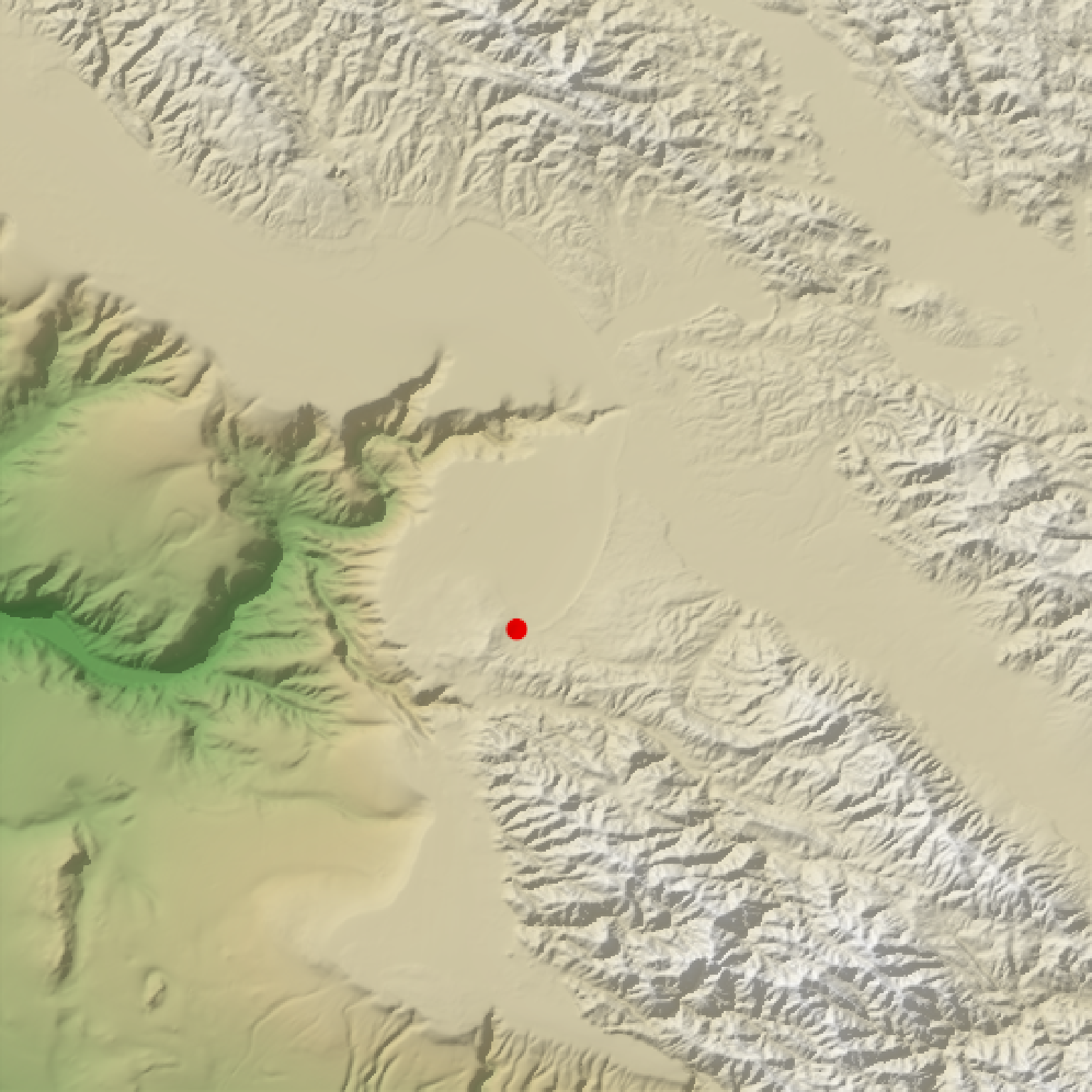Calculates and returns an overlay of points for the current map.

generate_point_overlay(
geometry,
extent,
heightmap = NULL,
width = NA,
height = NA,
pch = 20,
color = "black",
size = 1,
offset = c(0, 0),
data_column_width = NULL
)

## Arguments

geometry

An sf object with POINT geometry.

extent

Either an object representing the spatial extent of the scene (either from the raster, terra, sf, or sp packages), a length-4 numeric vector specifying c("xmin", "xmax","ymin","ymax"), or the spatial object (from the previously aforementioned packages) which will be automatically converted to an extent object.

heightmap

Default NULL. The original height map. Pass this in to extract the dimensions of the resulting overlay automatically.

width

Default NA. Width of the resulting overlay. Default the same dimensions as height map.

height

Default NA. Width of the resulting overlay. Default the same dimensions as height map.

pch

Default 20, solid. Point symbol. 0 = square, 1 = circle, 2 = triangle point up, 3 = plus, 4 = cross, 5 = diamond, 6 = triangle point down, 7 = square cross, 8 = star, 9 = diamond plus, 10 = circle plus, 11 = triangles up and down, 12 = square plus, 13 = circle cross, 14 = square and triangle down, 15 = filled square, 16 = filled circle, 17 = filled triangle point-up, 18 = filled diamond, 19 = solid circle, 20 = bullet (smaller circle), 21 = filled circle blue, 22 = filled square blue, 23 = filled diamond blue, 24 = filled triangle point-up blue, 25 = filled triangle point down blue

color

Default black. Color of the points.

size

Default 1. Point size.

offset

Default c(0,0). Horizontal and vertical offset to apply to the polygon, in units of geometry.

data_column_width

Default NULL. The numeric column to map the width to. The maximum width will be the value specified in linewidth.

## Value

Semi-transparent overlay with contours.

## Examples

#Add the included sf object with roads to the montereybay dataset
if(run_documentation()) {
monterey_city = sf::st_sfc(sf::st_point(c(-121.893611, 36.603056)))
montereybay %>%